## Introduction

The tremendous increase in complexity of infrastructural networks, like the Internet and those related with transportation and energy supply, is mandatorily accompanied by requirements of higher standards of system reliability, security and robustness. This trend can only be sustained if these complex networks have the right structure. Under this framework, the scale-free property, present in many real networks, determines important aspects related with their functionality1,2,3,4,5. However, while scale-free networks are usually quite robust against random failures, they typically break down rapidly under malicious attacks6,7,8,9,10,11. Numerical studies have recently revealed that this weakness can be mitigated if their structure becomes onion-like, which means that nodes of equal degree are connected among each other and to nodes of higher degree12,13. Since then, the properties of onion-like structures have been extensively investigated14,15,16,17,18,19,20,21,22,23. Based on this insight, here we will address the challenge of providing a paradigm for complex networks with better topology. More precisely, we show that it is possible to design a family of scale-free networks which are robust to random failures and considering some modifications, we can improve the resilience against malicious attacks. Additionally, these networks also exhibit other improved properties, like a finite shortest path and extreme sparseness in the thermodynamic limit, which substantially increases communication and reduces costs. Thus these new networks become potential candidates for the design and implementation of complex infrastructural networks.

In the deterministic network model introduced here, the nodes belonging to a given shell have intra-shell and inter-shell connections and the most connected nodes (hubs) are localized in the innermost shells. The network is recurrently expanded in such a way that every new generation corresponds to the addition of a new shell. Examples of these networks with four shells are shown in Figs. 1(a) and (b). Here we coin the name mandala network for this new family of graphs. In the first case, thereafter called network A, the first generation consists of a nucleus with three central nodes forming a complete graph (first shell). From each node in this nucleus, two new nodes emerge to form a connected ring of six nodes, composing the second shell of the second generation network. Following this iterative process, the third shell in the third generation network has an additional connected ring with twelve nodes, which, at this point, must also be linked to their respective ancestral nodes in the first and second shells. The same rules then apply for all new shells present in higher generation networks. This design therefore imposes that nodes at the same shell have the same degree. More precisely, the degree kig of a node at the i-th shell in the g-th network generation is given by,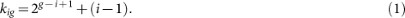Defining ni as the number of nodes in the i-th shell, by construction, we have that ni+ 1 = 2ni. From this relation, the number of nodes in the network is given by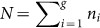, where the summation is over the total number g of shells.

In fact, the network described so far in Fig. 1(a) is a particular case resulting from the recursive method proposed here to generate an ensemble of scale-free networks. For example, in Fig. 1(b) we show another example of mandala network, thereafter named network B. Precisely, the method depends on a set of three parameters, (n1, b, λ), namely, the number of nodes in the first generation, n1, the number of new nodes added to each node in the more external shell, b and the scale factor, λ, for node degree in successive generations. Therefore, Eq. (1) can be written in a more general form as, kig = gi + (i − 1) and ni+ 1 = bni. For instance, the networks A and B are completely defined by the sets (3, 2, 2) and (4, 4, 2), respectively.

## Results

### Scale-free networks

Any network generated by this method has discrete degree spectrum. In order to characterize the scale-free dependence, we consider the cumulative degree distribution,. Taking into account that in each shell all nodes have the same degree, the cumulative distribution can be written as,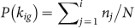. Applying Eq. (1) and the relation nj+ 1 = bnj, it can be shown that the cumulative distribution decays in the form, P(kig) ~ 1/kig. In Fig. 2, we show a logarithmic plot of the cumulative degree distribution for networks A and B. In both cases, we have the same scale-free dependence.

At this point, an explanation about the exponent of the degree distribution [p(k)] is useful24,25,26. Since our network has a discrete degree distribution, in order to calculate the standard definition of p(k), it becomes necessary to consider binned intervals between consecutive degrees. Thus, the degree distribution is calculated as, p(kig) ≡ ni/NΔkig, where Δkig = kigk(i + 1)g is the width of the interval. In this way, as Δkig ~ kig, it follows that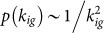.

### Ultra-small-world networks

Another important property of the mandala networks relates to the mean shortest path length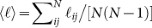, where ℓij is the shortest distance between any two nodes i and j in the network and the summation goes over all possible node pairs in the system. In our case, this expression can be written in a more convenient form as,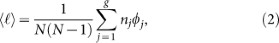where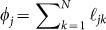is the sum of the shortest path lengths connecting a node in the j-th shell with all other nodes in the network, nj is the number of nodes in the j-th shell and the summation goes over the number of shells. Using the symmetry of the network A, for example, it is possible to show that ϕj = αjN − ξj (see the section Methods), where ξj has different values for different shells and αi is given by 5/3, 29/12, 5/2, 31/12, 63/24, for i = 1, 2, 3, 4 and 5, respectively, so that α → 8/3, for i. Taking into account the linear dependence of ϕj with N and considering the relations for nj, Eq. (2) reduces to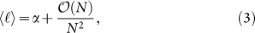which leads to 〈ℓ〉 → 8/3 in the thermodynamic limit, N. We show in Fig. 3 a semi-log plot of the mean shortest path length as a function of the number of nodes. The asymptotic convergence confirms our analytical result and therefore indicates that our network has an ultra-small-world behavior, namely 〈ℓ〉 becomes independent of N. One should note, however, that this result is still different from the case of a complete graph, for which 〈ℓ〉 = 1, corresponding to the mean-field limit. Applying a similar sequence of calculations to the network B, it can be readily shown that the mean shortest path length for this topology also converges to a constant in the limit of large system sizes, but now equal to 11/4.

### Highly sparse graphs

Next, we define the density d of connections as the ratio between the number of existing connections and the maximal number of possible connections for an undirected network with N nodes,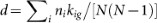. Considering the expression for kig given by Eq. (1), we can rewrite the definition of d in the following way: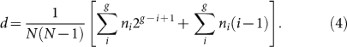Expressing both summations in Eq. (4) in terms of the number of nodes in the network,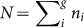and considering the limit of a very large number of generations, we obtain,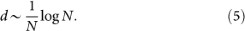The inset of Fig. 3 shows the dependence of the density of connections on the number of nodes for networks of type A, confirming the asymptotic behavior predicted by Eq. (5). In the case of network B, where the number of new nodes generated is twice that of network A, the density of connections decays faster. Indeed, applying the same approach and considering b = 4, it is possible to show for network B that d = (log N)/N. As a consequence, we conclude that our networks, despite of their ultra-small-world property, are extremely sparse when compared to the behavior of a complete graph, d = 1 and has only logarithmic correction to d ~ 1/N that is valid for the Erdös-Rényi network at the percolation threshold. It is worth noticing that mandala networks are reminiscent of the expander graphs27, since both models share similar properties as high sparsity and high connectedness. However, the mandala networks do not have self-loops or multiple edges with the same endpoints. Moreover, in the case of expander graphs, the maximal degree is limited, while in the mandala networks are scale-free.

### Robustness

The framework of percolation is usually considered for the analysis of the robustness of complex networks7,22,28,29,30,31,32,33,34. In this context, robustness is typically quantified by the critical fraction qc of removed nodes that leads to a total collapse of the network6,9,10,12. Nevertheless, as previously reported12,13,14,16, this approach does not account for situations in which the system can suffer a big damage without breaking down completely. The size of the giant component, the largest connected cluster in the system, during the removal process of nodes has been recently introduced12 as a refined measure to robustness,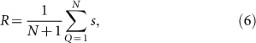where s is the fraction of nodes belonging to the giant component after removing Q = qN nodes, q is the fraction of nodes removed and R is in the range [0, 1/2]. The limit R = 0 corresponds to a system of isolated nodes, while R = 1/2 to the most robust network, which is the case of a completely connected graph. Here we check the robustness of our complex network model when subjected to mechanisms of random failures and two strategies of malicious attacks, namely, targeted by degree and targeted by betweenness6,7,8,9,10.

In the main plot of Fig. 4, we show the fraction s(q) of nodes belonging to the giant component during a random removal process as a function of the fraction of removed nodes q, for different values of the size N of networks A and B and averaged over 200 samples. Our results indicate that both networks A and B are robust, regardless of the system size N considered. This is corroborated in the inset of Fig. 4, where we plot the robustness measure R as a function of N for networks A and B. Both versions of the mandala network present a rather robust behavior, as compared to other models and real networks12,13, with R ≈ 0.45 and R ≈ 0.43, for types A and B, respectively.

Unfortunately, as originally defined, our model network does not present a resilient behavior when subjected to malicious strategies of attack. We first consider attacks whose targets are the surviving nodes with the highest degree. In our network, since nodes at the same shell have the same degree, we start by choosing a node randomly with equal probability from the set of nodes with the highest degree (first shell). Due to the hierarchical structure of the network, a node from the second shell will only be removed after all nodes from the first shell disappear. This removal sequence remains valid till the targeted attack reaches the second-last shell. At this point, the random removal of a node in this shell can cause the simultaneous disconnection of other nodes from the giant cluster in the same, as well as in the outermost shell. As shown in Fig. 5, this strategy of attack leads to a drastic collapse of the structure when we remove less than 40% of the nodes.

In order to improve the robustness of the mandala model to malicious attacks, we propose the following two types of modification on the original network structure. First, we can randomly rewire each edge of the last shell, maintaining invariant the density of connections. The results in Fig. 5 show that robustness increases to R = 0.36, as compared to the value R ≈ 0.30 of the original network. Second, as depicted in Fig. 5, we can systematically increase the number of connections between successive shells. As shown in Fig. 5, this change can promote a substantial increase in the resilience, depending on the number of additional connections per node, κ. In any case, it is important to notice that for all new versions considered, the obtained networks maintain their high-sparsity and ultra-small-world properties, since we just add a number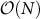of new links.

Another important type of targeted attack is to remove nodes sequentially according to their betweenness centrality, in a descending order. The original version of the mandala network also displays a fragile behavior when subjected to this type of process (see Fig. 6), collapsing before 10% of removal with R ≈ 0.027. Again, as Fig. 6 also shows, a rewiring process applied to the last shell can significantly improve the resilience of the mandala network, R = 0.32. This value is even larger than the one obtained for a Barabási-Albert network (γ = 3) having approximately the same density of connections (see Fig. 6).

### The Ising model

In small-world networks, the fact that the diameter of the graph does not grow faster than log N implies an infinite dimensionality. Mean-field theories therefore can successfully describe their critical behavior1,35,36,37,38,39. In order to investigate how collective ordering emerges in mandala networks, for which the shortest-path length is independent on N, we consider Ising spins σi associated to their nodes and ferromagnetic interactions J between them on the edges. Adopting the reduced Hamiltonian,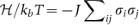, we perform Monte Carlo (MC) simulations on networks of type A for different system sizes N and temperature T values. In particular, we analyse the finite-size scaling properties of the model at the Tc = 0. The results in Fig. 7 show that the divergence of the maximum of the susceptibility with N, measured from the peak of the susceptibility, has the form,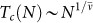, with a critical exponent,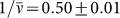.

## Discussion

In summary, we have presented a recursive method to generate an ensemble of complex networks defined by a set of three parameters, namely (n1, b, λ).

We have shown analytically and confirmed through numerical simulation that the networks originated from our model have scale-free topologies and are ultra-small, i.e., the average shortest-path lengths of sufficiently large networks become independent of their number of nodes. Our results also show that, as compared to a complete graph, which is ultra-small, these networks are highly sparse, with the density of edges going to zero with system size. Although mandala networks are robust against random failures, they are fragile against malicious attacks targeted by degree and betweenness centralities. However, simple corrections can be applied to the original model, improving significantly its robustness against both types of malicious attacks. Finally, we have verified that the critical temperature of the Ising model on the mandala network topology diverges with system size according to a power-law dependence, described by an exponent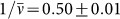. We expect to generalize this last result to other universality classes, for example, considering directed percolation and self-organized models on our deterministic networks.

## Methods

### The mean shortest path length

Consider the definition of the shortest path length: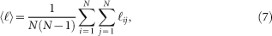and let us define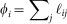as the summation of the shortest paths from the node i to all nodes in the network. Therefore, Equation (7) can be written as,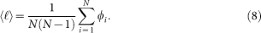From the symmetry of the network, nodes in the same shell have the same value of ϕ. Thus, we can interchange the summation in terms of the number of nodes to a summation in terms of the number of shells,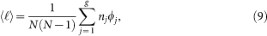where nj represents the number of nodes in the j-th shell and ϕj is now interpreted as the summation over the shortest paths from a node in the j-th shell to all nodes in the network.

To determine ϕj we choose a node, called root node, in the j-th shell and calculate its shortest path to other nodes, considering shell by shell. Here we will consider a detailed calculation for three first shells. For the first shell, considering the node 1 as root we obtain,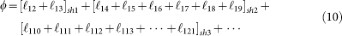The first bracket is equal to (n1 − 1), since all nodes in the first shell are at a distance equal to one for the root node. In the second shell, we have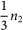nodes at a distance equal to one and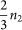nodes at a distance two of the root node. In this way, for the j-th outermost shells, we have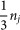nodes at a distance equal to one and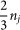nodes at a distance two of the root node. Using these results in Equation (10), it follows that,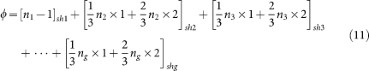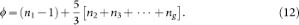Therefore, the value of ϕ for any node in the first shell is given by,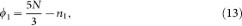where we consider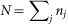. A similar approach can be applied to others shell. Choosing now the root node in the second shell, we obtain,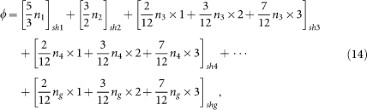and ordering the identical terms results in,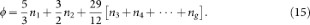The value of ϕ for nodes in the second shell is then given by,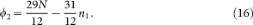Finally, using a similar sequence of calculation, for the i-th shell, we obtain that, ϕi = αiN − ξi, with αi equal to 5/3, 29/12, 5/2, 31/12, 63/24, 127/48 and 255/96 for the seven first shells, respectively. Therefore, we have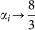for i.

### The Ising model

The Monte Carlo simulations of the Ising model on the mandala networks were performed using the Metropolis algorithm, starting from different initial spin configurations. In order to study the critical behavior of the system, we considered the magnetization ML and the susceptibility χL, which are defined by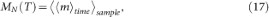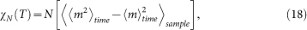where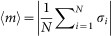, T is the temperature and N is the total number of nodes in the network. The symbols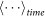and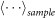, respectively, denote time averages taken in the stationary state and configurational averages taken over 100 independent samples. Time is measured in Monte Carlo steps (MCS) and 1 MCS corresponds to N attempts of changing the states of the spins. In our simulations, the initial 105 MCS were discarded to guarantee that the system reached the steady state, after which the time averages were estimated using the next 6 × 105 MCS. The value of temperature where χN has a maximum is identified as Tc(N) for N = 172, 684, 2732, 10924, 43692 and 174764.